# 卷积神经网络入门

### 目录

*以下所有图片援引自 Stanford CS231n

### CNN 结构概述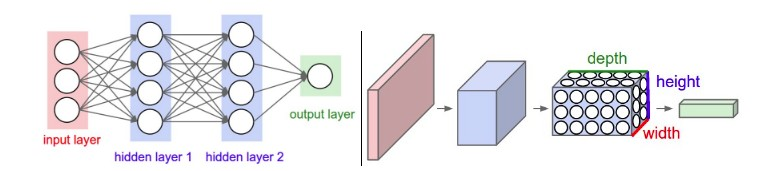### ConvNet 用到的各种网络层

• 输入层 [32×32×3] 是原始图像，长32，宽32，有3个色彩通道。
• 卷积层中每一个卷积核与输入层中的区域作点积，如果我们用8个卷积核，那么得到的结果就是 [32×32×8]。
• 激活层采用 ReLU 函数，它不会改变卷积的大小，结果依然是 [32×32×8]。
• 池化层进行了一个下采样操作，它不会改变深度，但是长与宽因为采样操作被缩小了，结果变成了 [16×16×8]。
• 全连接层用来计算结果，其中的每一个神经元都会与上层中的所有神经元相连，在 CIFAR-10 中，其结果变成了 [1×10]，表达了每种分类的置信度。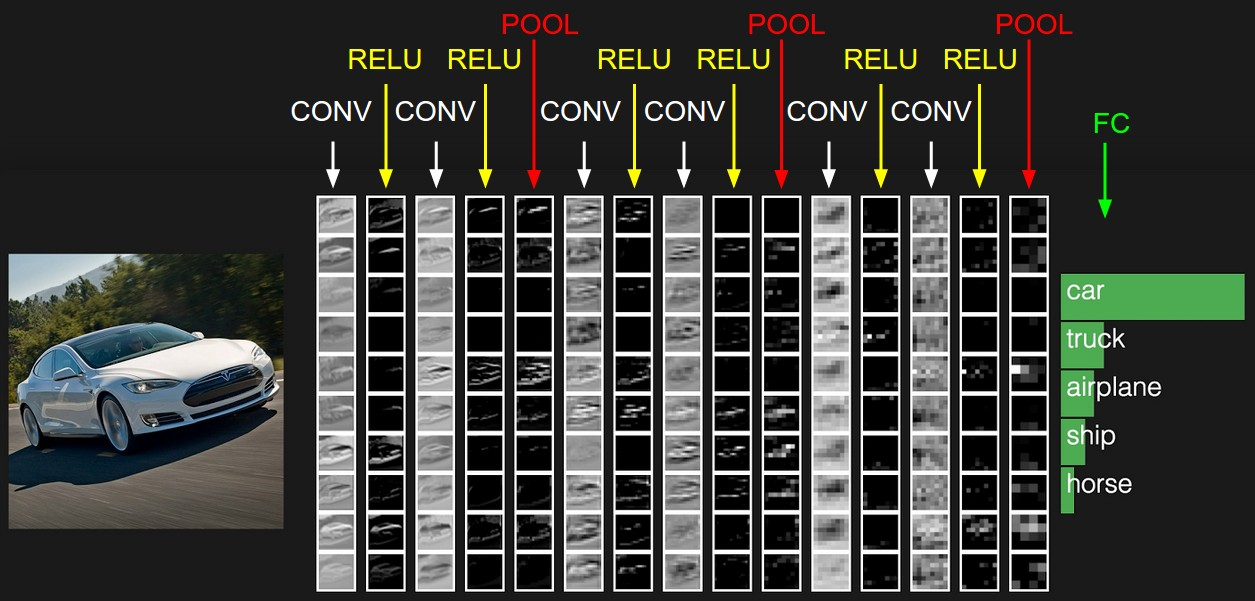#### Convolutional Layer 卷积层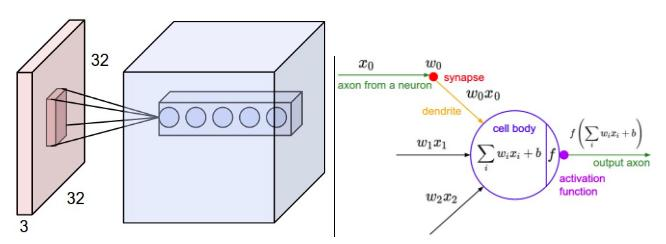• 卷积核个数决定了输出结果的深度，每一个深度上的神经元对原图都有着不同的相应（可能是边缘朝向，也可能是色彩偏好）。
• 步长指的是卷积核在输入上每次移动的距离。当步长为 1 时，每次移动 1 个像素，得到的结果和输入一样大（宽高）；当步长大于 1 时，得到的结果比输入小；当步长小于 1 时，得到的结果比输入大。
• 零填充指的是某些时候我们要在原图周围填充一些 0，这能方便我们调节平面的大小（通常遇到的情况是用这种方法使输入图片变成宽高相等）。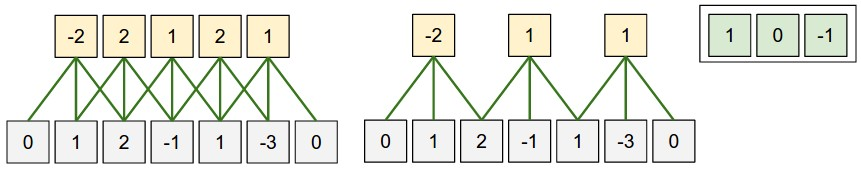#### Pooling Layer 池化层

• 输入卷积大小为 $$W_1 \times H_1 \times D_1$$
• 需要两个超参数：
• 区域大小(spatial extent) $$F$$
• 步长(stride) $$S$$
• 输出结果的大小为 $$W_2 \times H_2 \times D_2$$，其中：
• $$W_2=(W_1-F)/S+1$$
• $$H_2=(H_1-F)/S+1$$
• $$D_2=D_1$$
• 不具有学习性，没有响应的参数
• 一般在池化层中不使用零填充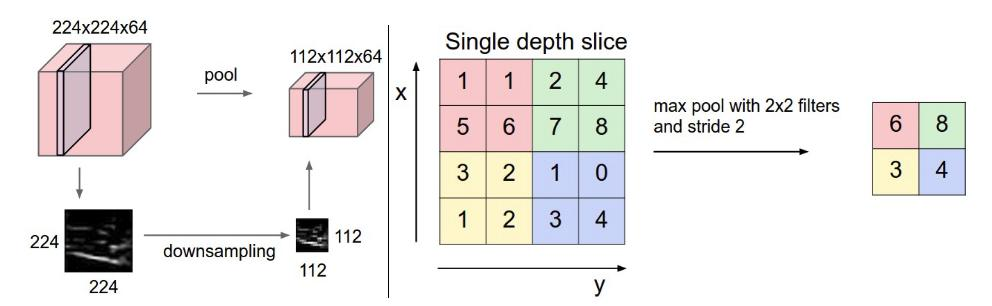### ConvNet 常见架构

#### 层级结构

Input → [Conv → ReLU]*N → Pool?]*M → [FC → ReLU]*K → FC

• LeNet.
• AlexNet.
• ZF Net.